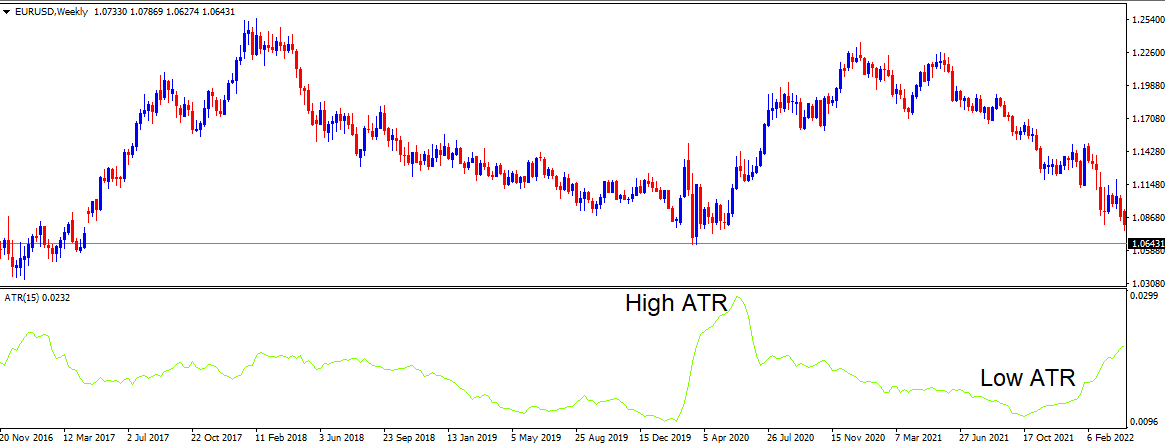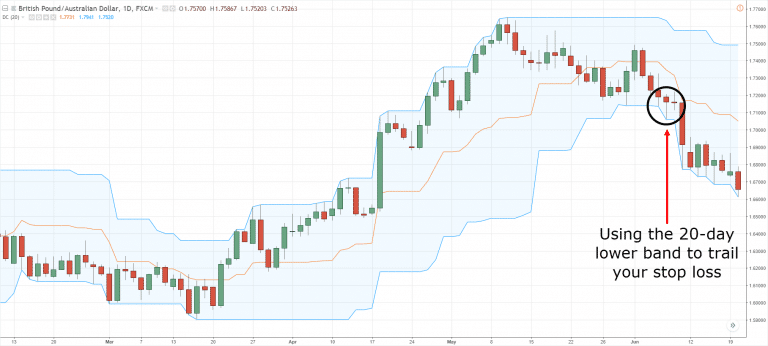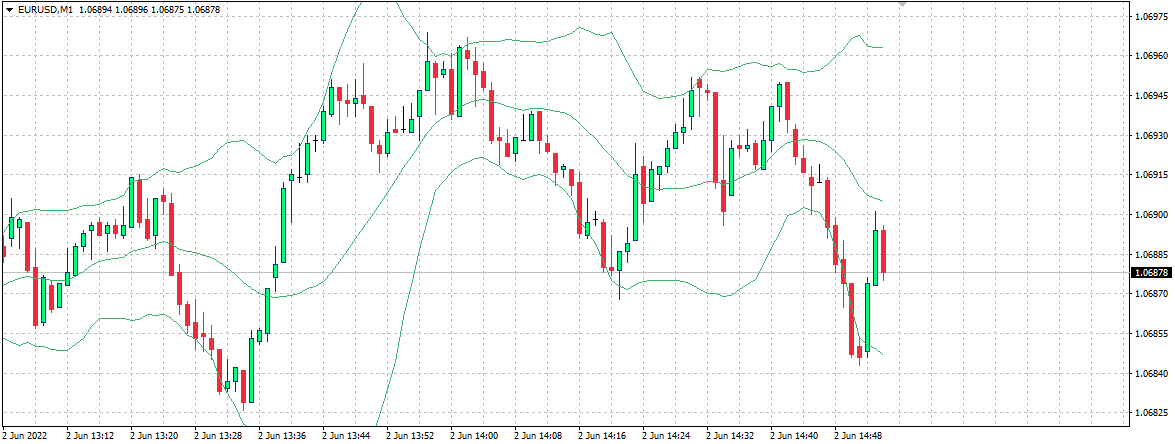Tara Visser June 21, 2022 Average reading time: 5 min

# Volatility

Anytime you look at live forex quotes, the prices are always changing. This can also be seen in the forex charts as the patterns go up and down with time. Sometimes, the price fluctuations move rapidly up and down. This is known as high volatility but when the price is stable without much fluctuation, it is low volatility or high liquidity.

In the forex market, Volatility is a measure of the rate at which the price of a currency pair moves with time. It is a way of describing how large or small the exchange change varies with time. In the forex charts, the bigger candlesticks indicate more volatility than the smaller candlesticks.

Volatility is also used when comparing the price movements of two quantities such as currency pairs. For example; the GBPUSD is generally more volatile than the EURUSD. This is because looking at daily or weekly charts of the two currency pairs; it shows that GBPUSD moves more than EURUSD.

Understanding and detecting volatility is important in forex trading because it provides trading opportunities with high-profit potentials; although it comes with more risk. Technical analysts are much interested in trends, momentum, and volatility. Learning how to measure these variables will help you make more accurate predictions and ultimately more profits.

## Rules of volatility

• Volatility clustering: This was first observed by Mandelbrot in 1963. He observed that large changes are likely to be followed by large changes and small changes also tend to be succeeded by small changes. In the forex markets, it has been observed that volatility that will occur tomorrow is often similar to or close to the volatility of today. If a forex pair moves 100 pips today, it may probably move around the same 100 pips tomorrow. In effect, if a forex pair moves 60 pips today, the probability of moving by 150 pips tomorrow is slim.
• When relatively low volatility is experienced for the long term, then, when the volatility will be high when it eventually starts. For example, when the prices of forex pairs are stable over weeks, the directional breakout is likely to be strong when it comes.
• When there has been liquidity or price stability for a while, it is best to open a trade position when the volatility begins. This rule is derived from a combination of the first two rules above.

## Volatility measurement

Some technical indicators are used to measure volatility. These indicators can help the trader to find profitable trades. Below are some of them:

### Average True Range (ATR)

This indicator shows the average trading range of the forex pair for a specified period. The trader chooses the period while the ATR takes the price range, that is, the difference between the low and high for each time frame. It then plots a moving average using the range obtained for each time frame. Depending on the price, ATR may use also use the difference between the previous candle’s closing price and the current candle’s high price.

So, the ATR for a period of 15 on an H1 will be calculated by taking the price range for the last 15 hours and plotting moving averages with the values.

Consider the chart below, notice that increased volatility showed rising ATR and decreased volatility shows low ATR values. ATR does not measure trend direction, momentum, support, or resistance levels.Sometimes, a trader may use 2 ATRs with different periods in one chart to further identify if recent volatility is high or low.

### Donchian Channels

This is a well-known technical indicator developed by Richard Donchian. It is used to determine volatility in the prices of a forex pair and also to find potential breakouts and retracements. Donchian channels comprise 3 bands: the upper band, and middle and lower bands.

Donchian channels is calculated for n period as follows:

• The upper band is the highest price in the last n periods
• Middle band = (Upper band + Lower band ) / 2
• The lower band is the lowest price in the last n periods.In times of low volatility, market movements are small, therefore the Donchian channel will be narrow but in times of high volatility, the channels are wide. Also, if the price of the forex pair goes above the middle band, it may signal a bulling trend prompting traders to open a long position until it hits the upper band. But, if the price drops below the middle band, it may likely mean a bearish trend, and traders can short sell the forex pair.

### Bollinger bands

Similar to Donchian Channels, Bollinger bands use 3 channels, which also widen in times of high volatility and narrow in times of low volatility. But Bollinger bands are developed by John Bollinger and they use standard deviations.The middle band is a simple moving average plotted with a period inputted by the trader; the upper and lower channels are standard deviations above and below the simple moving average price. In an uptrend, the price moves to the upper band and crosses it if the trend is strong. The reverse is the same for a strong downtrend.

### Linear Regression Indicator

Linear regression is used in statistics to analyze two separate variables in order to form a single relationship. In forex trading, the variables of price and time are analyzed along a normal distribution to identify a resistance or support zone.

The Linear Regression technical indicator plots a linear regression of the closing prices of the forex pair for a defined period specified by the trader. It generally shows where the price is supposed to be at any given time. The indicator consists of 3 channel lines; the middle channel is the linear regression of the prices which can be set to be opening, closing, high or low. The two upper and lower channels are the high and low standard deviations above and below the regression line.

As usual, areas, where the channel is wide, indicate high volatility, and low volatility is indicated by narrow channel areas.

## Conclusion

Volatility refers to the rapid movement of the prices of a forex pair. In times of high volatility, prices go up and down rapidly but in times of low volatility, prices are more stable. The rules of volatility say that small changes follow each other and likewise big price changes. Long periods of low volatility may be followed by a spike when it is time for high volatility and it is best to open trades when the changes start.

ATR indicators, Donchian Channels, Bollinger bands, and Linear Regression indicators are some of the popular indicators used to measure price volatility in the forex market.